# 6.1 引言

• 现实中存大量聚类问题

• 聚类分析目的
• 把对象按一定规则分成若干类,类不事先给定,
• 而是根据数据特征确定,对类的数目和类的结构不作任何假定。
• 同类里的对象相似,不同类里的对象不相似。
• 聚类分析常探索寻找“自然的”或“实在的”分类,
• 这样的分类应是对所研究的问题有意义的。
• 聚类分析也能够用来概括数据。

• 判别分类和聚类分析
• 都是研究事物分类(或组)的基本法
• 区别:
• 组数目已知,将样品分配给事先已定义好的组(或类)之一;
• 类数目还是类本身在事先都是未知。
• 联系:如果组不是已有,则对组的事先了解和形成有时可通过聚类分析探索;
• 聚类分析的效果往往也可以通过由前两个(或三个)费希尔判别函数得分产生的散点图(或旋转图)从直觉上评估。

• 根据分类对象不同
• Q:对样品的聚类,
• R:对变量的聚类。
• 本章主Q

# 6.2距离和相似系数

• 对样品(变量)分类,样品(或变量)相似性咋度量?
• 两相似性度量:距离和相似系数
• 距离是不相似性的度量。

• 距离和相似系数有不同的定义,
• 这些定义与变量类型有关
• 间隔变量:变量用连续量表示,如长度
• 有序变量:变量度量时不用明确的数量表示,用等级表示,
• 如某产品分一等、二等、三等
• 名义变量:变量用一些类表示,
• 类之间无等级也无数量关系,
• 性别、职业、产品号

• 间隔变量也称定量变量,
• 有序和名义
• 统定性变量
• 属性变量
• 分类变量

• 对间隔变量,
• 距离度量样品之间相似性,
• 相似系数常度量变量间相似性
• 相似系数也
• 度量基于有序或名义变量的样品间相似性。
• 本章基于间隔变量的样品聚类分析方法

## 一 距离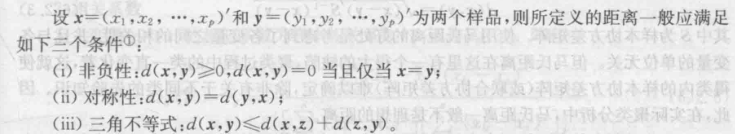• 聚类过程中,相距较近的样品点归一类,
• 较远的样品点应属不同类。
• 常用的距离有如下几:

• Minkowski距离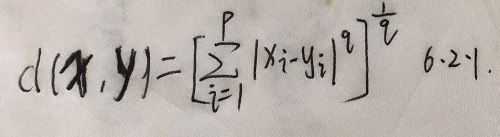• $q\ge 1$
• $q=1$时,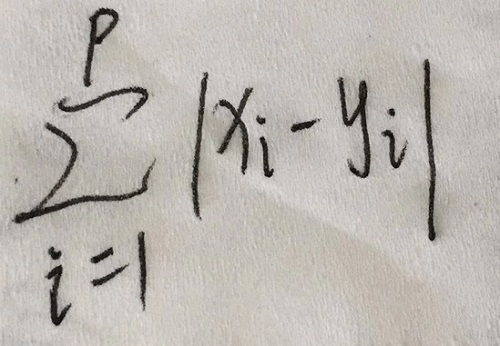• 绝对值距离,形象称“城市街区”距离
• 当城市街区中位置点之间的远近用路程来度量时
• 用绝对值距离( $p=2$)比较合适
• $q=2$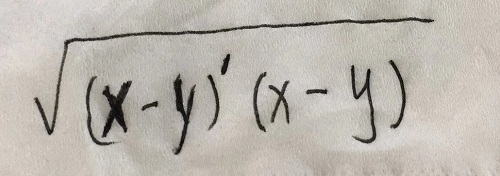• 欧氏距离,聚类分析中最常用的距离

• $q=\infty$,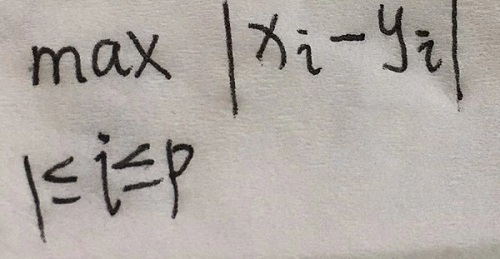• 切比雪夫距离

• 欧氏距离对(大的)异常值敏感,
• 绝对值距离却对异常值相对不太敏感。
• $q$越大,差值大的变量在距离计算中起的作用就大,
• 对异常值越敏感。

• 各变量的单位不同或变异性相差很大时
• 不直接用明氏,先对各变量的数据作标准化处理,
• 用标准化后的数据计算距离。
• 最常用的标准化处理是,令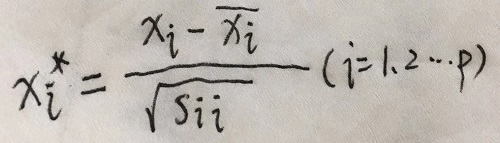• $x_i$的样本均值和样本方差。

• 兰氏距离
• 当数据皆为正,兰氏距离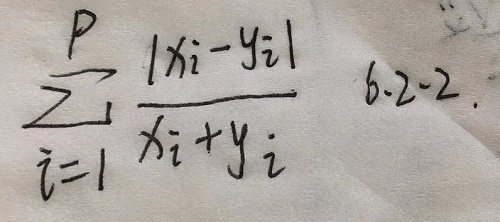• 距离与各变量单位无关,
• 适用于高度偏斜或含异常值的数据

• 3.马氏距离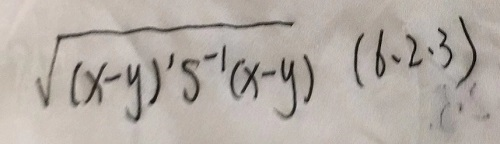• $S$样本协方差矩阵。
• 考虑各变量间相关性,与各变量单位无关
• 缺陷,聚类过程中的类一直变化,使类内的样本协方差矩阵(或联合协方差矩阵)难确定,除非有关于不同类的先验知识。
• 实际聚类分析中,马氏不是理想的

• 以上几种要求变量是间隔尺度,
• 如果变量是有序尺度或名义尺度
• 则有相应的一些定义样品之间距离和相似系数的方法。
• 下例给出对二值名义变量的一种简单距离定义

• 例6.2.1

• 学员的资料中得到这样六个变量

• $x_1$:性别(男,女)

• $x_2$:外语语种(英语,非英语)

• $x_3$:专业(统计,非统计)

• $x_4$:职业(教师,非教师)

• $x_5$:居住处(校内,校外)

• $x_6$:学位(硕士,学士)

• 两名:

• $x$=(男,英语,统计,非教师,校外,学士)

• $y$=(女,英语,非统计,教师,校外,硕士)

• 记配合的变量数为 $m_1$,不配合 $m_2$

• 则距离定义为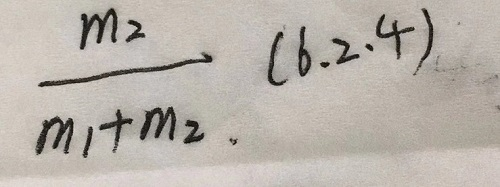• 本例距离为2/3

## 二 相似系数

• 对变量聚类时,常用相似系数作为变量间相似性度量。
• 要看大小,另一些看相似系数绝对值大小
• 相似系数(其绝对值)越大,变量间相似性程度越高
• 聚类时,较相似的变量归一类,
• 不太相似的变量属不同类。
• 变量 $x_i$ $x_j$的相似系数用 $c_{ij}$
• 一般满足: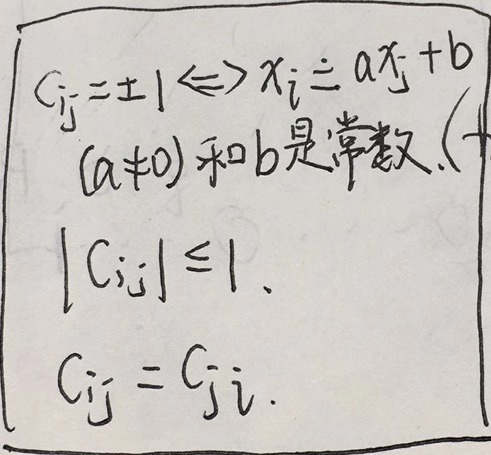• 常用的相似系数有如下

• 夹角余弦
• $\theta_{ij}$ $R^n$
• 变量 $x_i$的观测向量与
• 变量 $x_j$的观测向量间的夹角
• 定义俩变量相似系数为 $\cos \theta_{ij}$
• 记作 $c_{ij}(1)$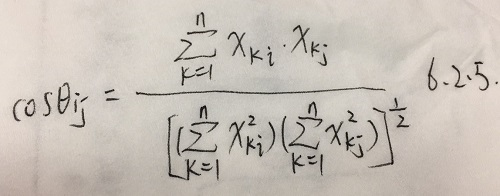• 相关系数
• 变量与变量的相似系数
• 为样本相关系数 $r_{ij}$,记作 $c_{ij}(2)$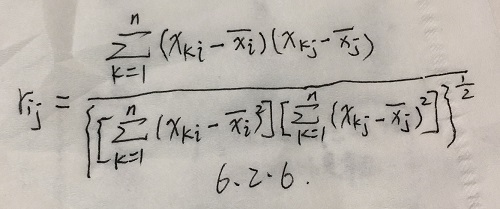• 如果变量 $x_i$ $x_j$皆已标准化了
• 则它们间的夹角余弦就是相关系数

• 相似系数除有时也度量样品间的相似性
• 距离有时也度量变量间相似性
• 由距离来构造相似系数总是可能的,令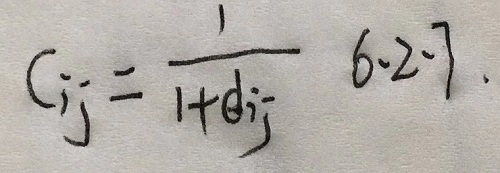• d为第 $i$个样品与第 $j$的距离,
• $c_{ij}$可作为相似系数,来度量样品间相似性
• 然而距离须满足三条件,不总能由相似系数构造。
• 高尔( Gower)证明
• 当相似系数矩阵 $(c_{ij})$非负定时,如令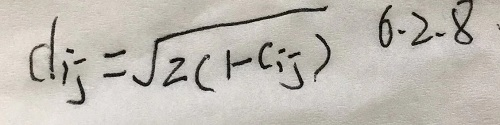• $d_{ij}$满足三条件

• 同一批数据用不同的相似性度量,得到不同分类结果
• 聚类分析中,应根据实际情况选取合适的相似性度量。
• 经济变量分析中,常用相关系数来描述变量间的相似性程度。